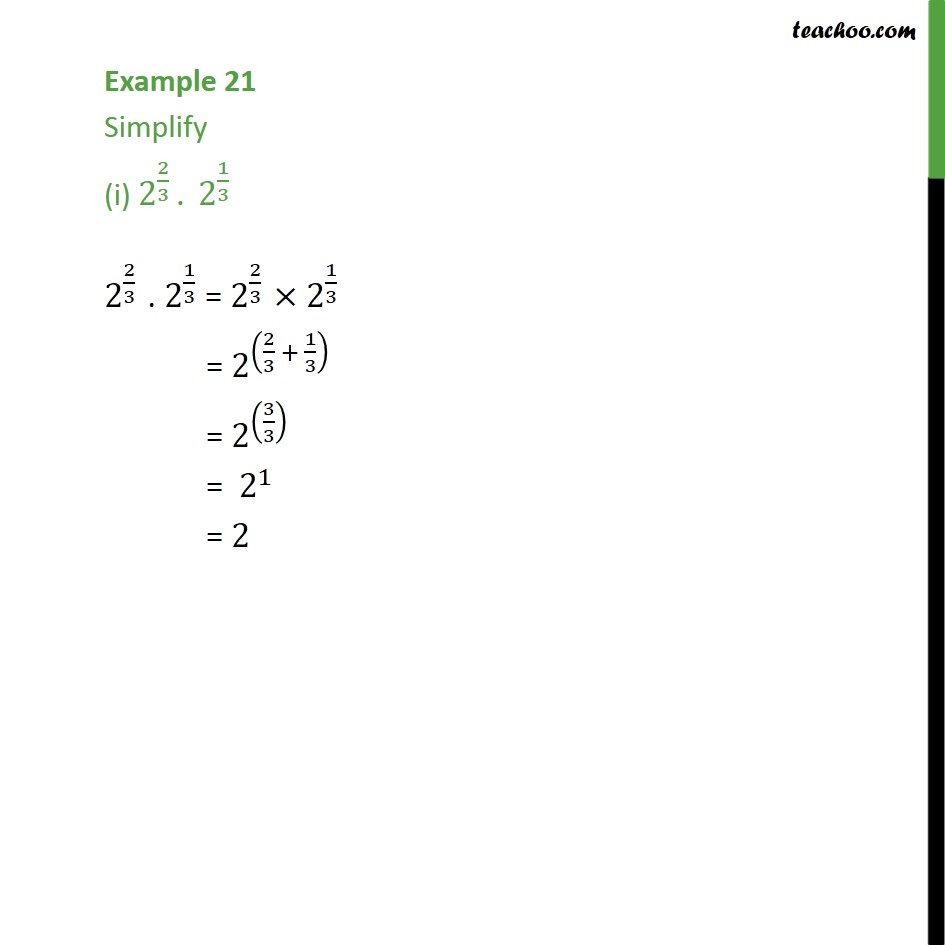1. Chapter 1 Class 9 Number Systems (Term 1)
2. Concept wise
3. Laws of exponents

Transcript

Example 21 Simplify (i) 2^(2/3) . 2^(1/3) 2^(2/3) . 2^(1/3) = 2^(2/3) × 2^(1/3) = 2^((2/3 + 1/3) ) = 2^((3/3) ) = 〖 2〗^1 = 2